Q.1) Express the amount of money in given terms ?
3 rupees 85 paise = ₹ ?
a) ₹ 3.085
b) ₹ 3.85

Q.2) Convert:
3 rupees = ___ paise
a) 30 paise
b) 300 paise

Q.3) Convert:
400 paise = ___ rupees.
a) ₹ 4
b) ₹ 40

₹ 300 and ₹ 500
a) ₹ 900
b) ₹ 800
c) ₹ 1000

₹ 41.35 and ₹ 23.21
a) ₹ 64.56
b) ₹ 62.16
c) ₹ 68.16

Q.6) Subtract:
₹ 864 – ₹ 153
a) ₹ 723
b) ₹ 699
c) ₹ 711

Q.7) Subtract the following:
₹ 84.98 and ₹ 33.54
a) ₹ 53.56
b) ₹ 51.44
c) ₹ 55.04

### Money Worksheets Grade 3 Explanations

Q.1) Explanation – Money Worksheets Grade 3

Whenever, we are given both Rupees and paise, and we need to write them in rupees, we convert the given paisa into Rupees by adding a dot ( . ) after rupees value , to separate rupees and paise.
Paise are always written as a two-digit number.
3 rupees 85 paise = ₹ 3.85

Correct Answer – b) ₹ 3.85

Q.2) Explanation – Money Worksheets Grade 3

As we know that,
1 rupees = 100 paise To find 3 rupees in paise .
We have to multiply both sides by 3
3 rupees = ( 3 x 100 ) paise
3 rupees = 300 paise

Correct Answer – b) 300 paise

Q.3) Explanation – Money Worksheets Grade 3

As we know that,
₹ 1 = 100 paise
or 100 paise = ₹ 1
then,
1 paise = ₹ 1/100
To find 400 paise in rupees. . We have to multiply both sides by 400
( 400 x 1 ) paise = ₹ ( 400 x 1/100 )
400 paise = ₹ 4

Q.4) Explanation – Money Worksheets Grade 3

Since both the values contain only Rupees ( ₹ ), we would add the values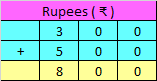Hence, ₹ 300 + ₹ 500 = ₹ 800

Correct Answer – b) ₹ 800

Q.5) Explanation

Step I: Write Rupees ( ₹ ) and Paise ( p ) aligned in the rupees and paise columns.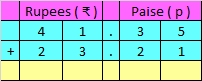Step II: Add Rupees ( ₹ ) and Paise ( p ) as we add numbers in Addition.

We would add the numbers in a normal way, as we do addition, starting from the right side, i.e paise ​side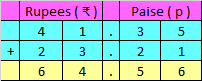Hence, the sum is ₹ 64.56

Correct Answer – a) ₹ 64.56

Q.6) Explanation –

Since both the values contain only Rupees ( ₹ ), we would subtract the values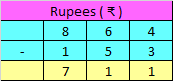Hence, ₹ 864 – ₹ 153 = ₹ 711

Correct Answer – c) ₹ 711

Q.7) Explanation – Money Worksheets Grade 3

Step I: Write Rupees ( ₹ ) and Paise ( p ) aligned in the rupees and paise columns.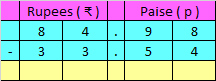Step II: Subtract Rupees ( ₹ ) and Paise ( p ) as we subtract numbers in Subtraction.

We would subtract the numbers in a normal way, as we do in subtraction, starting from the right side, i.e paise side.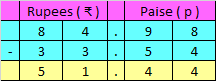Hence, the difference is ₹ 51.44

Correct Answer – b) ₹ 51.44